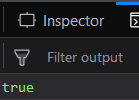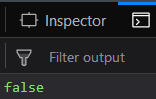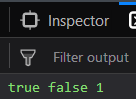# Underscore.js | _.isRegExp() with Examples

_.isRegExp() function:

• It finds whether the object passed is a regular expression or not.
• If the object is a regular expression then it returns true otherwise false.
• We can even apply operations like addition etc on the variables in which the result of _.isRegExp() is stored.

Syntax:

`_.isRegExp(object)`

Parameters: It takes only one argument which is the object that needs to be checked.

Return value: It returns true if the object passed is a regular expression and if not then false is returned.

Examples:

1. Passing a regular expression to the _.isRegExp() function: The _.isRegExp() function takes the element from it’s parameter and starts checking if it is a regular expression or not. Since the object starts and ends with ‘/’, therefore it is a regular expression. Hence, the result is true.

 `<``html``> ` `   `  `<``head``> ` `    ``<``script` `src` `=  ` `"https://cdnjs.cloudflare.com/ajax/libs/underscore.js/1.9.1/underscore-min.js"` `> ` `    `` ` ` ` `   `  `<``body``> ` `    ``<``script` `type``=``"text/javascript"``> ` `        ``console.log(_.isRegExp(/geek/)); ` `    `` ` ` ` `   `  ` `

Output:2. Passing a string to the -.isRegExp() function: In this we are passing a string to the _.isRegExp() and this can be identified as the parameter passed is inside the ‘ ‘ (quotes). Since a string is not a regular expression therefore, the output will be false.

 `<``html``> ` `   `  `<``head``> ` `    ``<``script` `src` `=  ` `"https://cdnjs.cloudflare.com/ajax/libs/underscore.js/1.9.1/underscore-min.js"` `> ` `    `` ` ` ` `   `  `<``body``> ` `    ``<``script` `type``=``"text/javascript"``> ` `        ``console.log(_.isRegExp('geek')); ` `    `` ` ` ` `   `  ` `

Output:3. Passing a string with ‘/’ to _.isRegExp() function: The _.isRegExp() function takes the parameter which in this case is inside ‘ ‘, hence it is a string. Therefore, all the letters, symbols inside ‘ ‘ will behave as a string character. Hence the overall object is a string. Therefore, the output is false.

 `<``html``> ` `   `  `<``head``> ` `    ``<``script` `src` `=  ` `"https://cdnjs.cloudflare.com/ajax/libs/underscore.js/1.9.1/underscore-min.js"` `> ` `    `` ` ` ` `   `  `<``body``> ` `    ``<``script` `type``=``"text/javascript"``> ` `        ``console.log(_.isRegExp('/geek/')); ` `    `` ` ` ` `   `  ` `

Output:4. Applying addition operation on the _.isRegExp() function’s output:
In this we are storing the result of both the example 1 and 2 in the variables ‘a’ and ‘b’. Then we are applying addition operation on both the ‘a’ and ‘b’ variables. Since ‘a’ is true and ‘b’ is false, therefore, the addition of true and false will result in 1 which is then stored in ‘c’ variable.

 `<``html``> ` `   `  `<``head``> ` `    ``<``script` `src` `=  ` `"https://cdnjs.cloudflare.com/ajax/libs/underscore.js/1.9.1/underscore-min.js"` `> ` `    `` ` ` ` `   `  `<``body``> ` `    ``<``script` `type``=``"text/javascript"``> ` `        ``var a=_.isRegExp(/geek/); ` `        ``var b=_.isRegExp('geek'); ` `        ``var c=a+b; ` `        ``console.log(a, b, c); ` `    `` ` ` ` `   `  ` `

Output:`` `<``script` `type``=``"text/javascript"` `src` `= ` `"https://cdnjs.cloudflare.com/ajax/libs/underscore.js/1.9.1/underscore-min.js"``> ` ` `My Personal Notes arrow_drop_upCheck out this Author's contributed articles.

If you like GeeksforGeeks and would like to contribute, you can also write an article using contribute.geeksforgeeks.org or mail your article to contribute@geeksforgeeks.org. See your article appearing on the GeeksforGeeks main page and help other Geeks.

Please Improve this article if you find anything incorrect by clicking on the "Improve Article" button below.

Improved By : shubham_singh